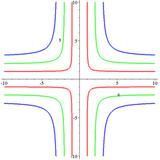Cruciform Curve

# Cruciform curve

to get instant updates about 'Cruciform Curve' on your MyPage. Meet other similar minded people. Its Free!

X

Description:
The cruciform curve, or cross curve is a quartic plane curve given by the equation

[itex]x^2y^2-b^2x^2-a^2y^2=0 ,[/itex]

where a and b are two parameters determining the shape of the curve.The cruciform curve is related by a standard quadratic transformation, x ↦ 1/x, y ↦ 1/y to the ellipse a<sup>2</sup>x<sup>2</sup> + b<sup>2</sup>y<sup>2</sup> = 1, and is therefore a rational plane algebraic curve of genus zero. The cruciform curve has three double points in the real projective plane, at x=0 and y=0, x=0 and z=0, and y=0 and z=0.

Because the curve is rational, it can be parametrized by rational functions. For instance, if a=1 and b=2, then
[itex]x = -frac, y = frac[/itex]
parametrizes the points on the curve outside of the exceptional cases where the denominator is zero.

No feeds found

AllPosting your question. Please wait!...